# Intermediate Geometry : How to find if acute / obtuse triangles are congruent

## Example Questions

### Example Question #82 : Triangles

Given: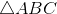and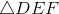.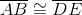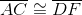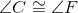True or false: It follows from the given information that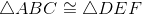.

False

True

False

Explanation:

Examine the diagram below.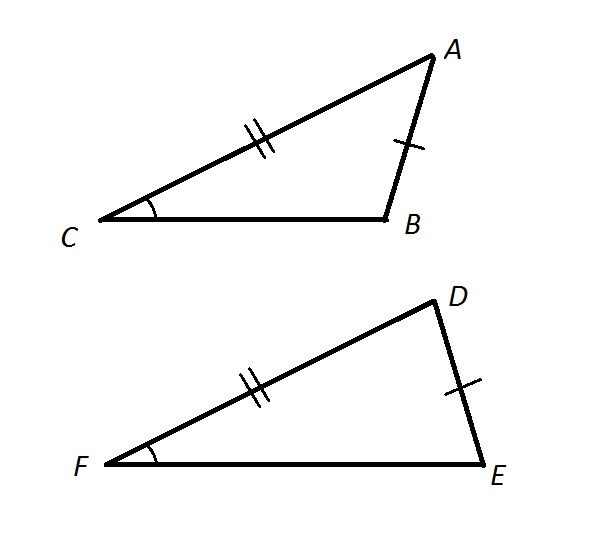,, and, but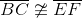. As a result, it is not true that. Therefore, the statement is false.

### Example Question #1 : How To Find If Acute / Obtuse Triangles Are Congruent

Given:and.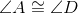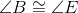True or false: It follows from the information given that.

True

False

False

Explanation:

The congruence of corresponding angles of two triangles does not alone prove that the triangles are congruent. For example, see the figures below: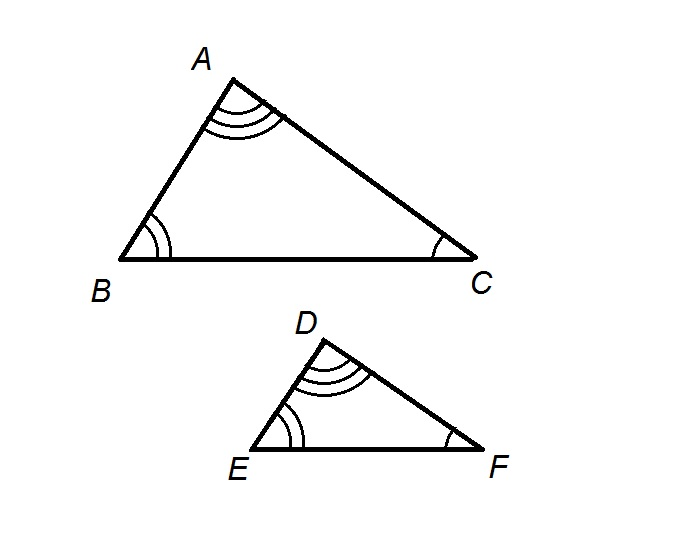The three angle congruence statements are true, but the sides are not congruent, so the triangles are not congruent. The statement is false.

### Example Question #84 : Triangles

Given:and.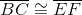True or false: It follows from the given information that.

False

True

True

Explanation:

As we are establishing whether or not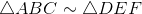, then, andcorrespond respectively to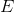, and.

By the Side-Side-Side Congruence Postulate (SSS), if all three pairs of corresponding sides of two triangles are congruent, then the triangles themselves are congruent. Betweenand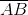and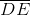are corresponding sides, their congruence is given. The other two congruences between corresponding sides are given, so the conditions of SSS are satisfied.is indeed true.

### Example Question #85 : Triangles

Given:and.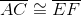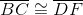True or false: It follows from the given information that.

True

False

False

Explanation:

As we are establishing whether or not, then, andcorrespond respectively to, and.

By the Side-Side-Side Congruence Postulate (SSS), if all three pairs of corresponding sides of two triangles are congruent, then the triangles themselves are congruent. However, if we restate the first side congruence as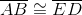and examine it with the other two:We see that while we can invoke SSS, the points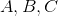correspond to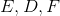, respectively. The triangle congruence that follows is therefore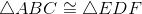.

### Example Question #86 : Triangles

Given:and.True or false: It follows from the given information that.

False

True

True

Explanation:

As we are establishing whether or not, then, andcorrespond respectively to, and.

By the Side-Angle-Side Congruence Postulate (SAS), if two pairs of corresponding sides and the included angle of one triangle are congruent to the corresponding parts of a second, the triangles are congruent.and, indicating congruence between corresponding sides, and, indicating congruence between corresponding included angles. This satisfies the conditions of SAS, sois true.

### Example Question #87 : Triangles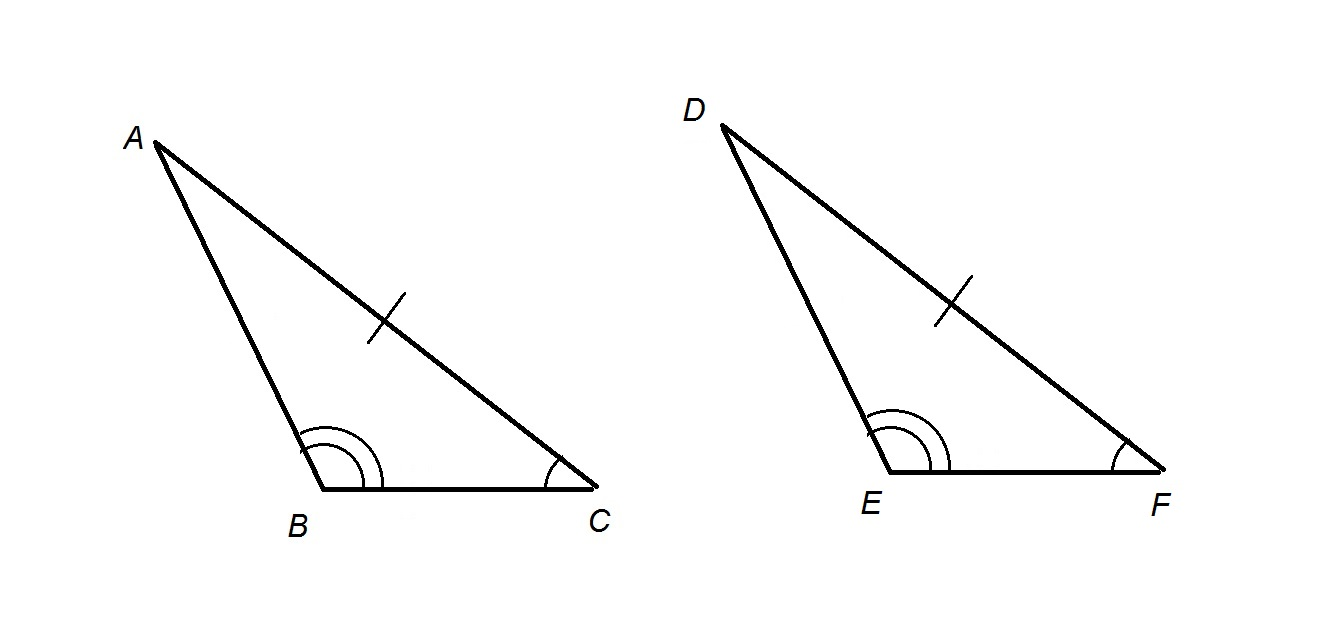Refer to the above two triangles. By what statement does it follow that?

The Converse of the Pythagorean Theorem

The Hinge Theorem

The Angle-Angle-Side Theorem

The Angle-Side-Angle Postulate

The Converse of the Isosceles Triangle Theorem

The Angle-Angle-Side Theorem

Explanation:

We are given that two angles of-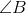and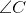-  and a nonincluded side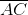are congruent to their corresponding parts,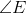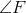, and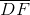of. It follows from the Angle-Angle-Side Theorem that.

### Example Question #88 : Triangles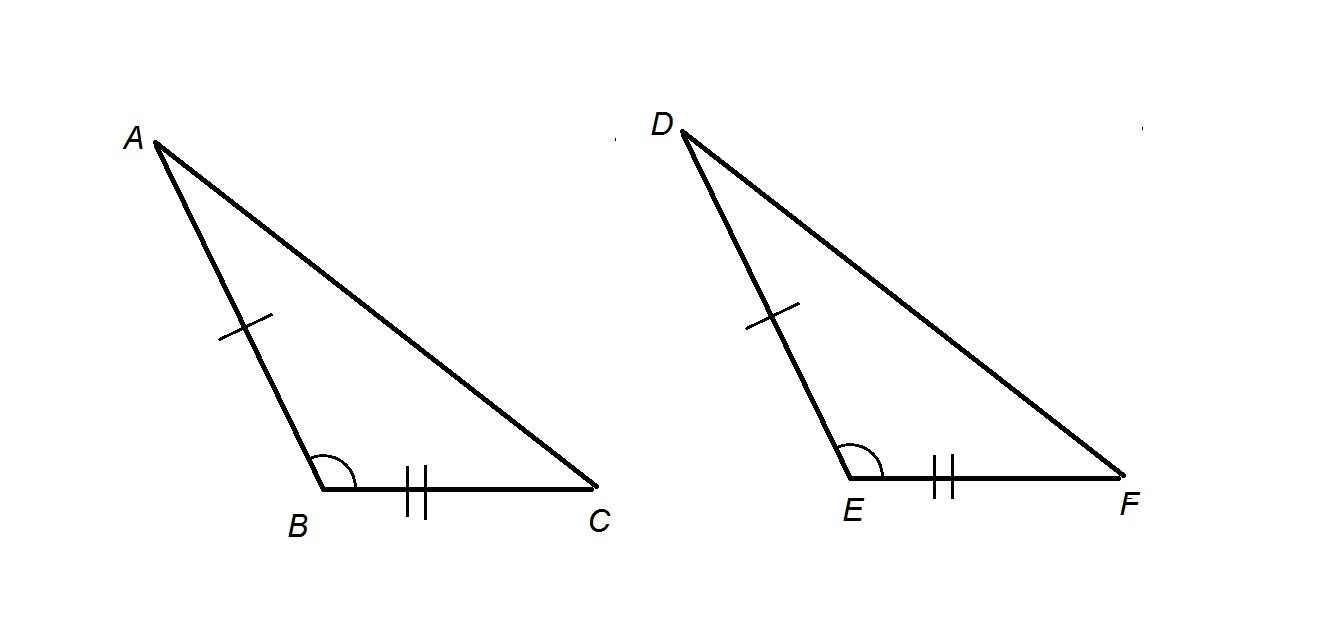Refer to the above two triangles. By what statement does it follow that?

The Side-Angle-Side Postulate

The Isosceles Triangle Theorem

The Triangle Midsegment Theorem

The Angle-Angle Postulate

The Hinge Theorem

We are given that two sides of- sidesand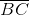- and their included angleare congruent to their corresponding parts, sidesand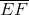andof. It follows from the Side-Angle-Side Postulate that.